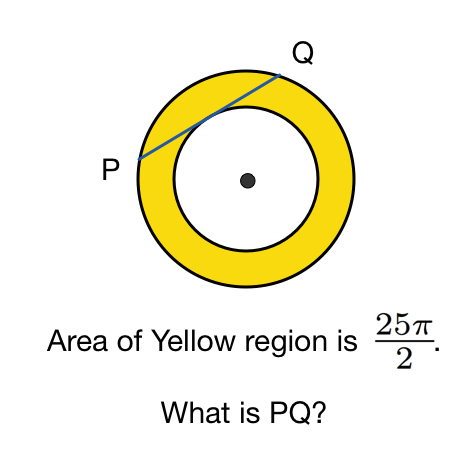# Inside And Outside

Geometry Level 2The above shows an annulus, a region bounded by two circles sharing the same center. straight line $PQ$ is a tangent to the circumference of the small circle and is a chord of the big circle.

If the area of annulus is $\dfrac{25\pi}2$, find the length $PQ$.

×